# Bluetooth LE Direction Finding for Tracking Node Position

This example shows you how to track the 2-D or 3-D position of a Bluetooth® low energy (LE) node by using Bluetooth® Toolbox.

Using this example, you can:

• Simulate direction-finding packet exchange in the presence of radio frequency (RF) front end impairments, path loss, and additive white Gaussian noise (AWGN).

• Track the node position by using Bluetooth direction-finding features and position estimation techniques.

• Improve the location accuracy by using a Kalman filter from Sensor Fusion and Tracking Toolbox™.

### Bluetooth LE Direction Finding

The Bluetooth Core Specification v5.1  introduced new features that support high-accuracy direction finding. Bluetooth direction finding presents two distinct methods to estimate the 2-D or 3-D location of the Bluetooth LE node: angle of arrival (AoA) and angle of departure (AoD). In AoA and AoD techniques, the antenna array is present at the receiver and transmitter, respectively. Some commonly used antenna arrays are uniform linear array (ULA) and uniform rectangular array (URA). For more information on Bluetooth direction finding, see Bluetooth Location and Direction Finding.

### Location Finding Methods

The example uses these terminologies:

• Node - The device whose location is to be determined.

• Locator - The receiving device (in AoA calculation) or transmitting device (in AoD calculation).

This figure shows two different ways to calculate the location of the Bluetooth LE node. Each circle indicates the range of a Bluetooth LE locator.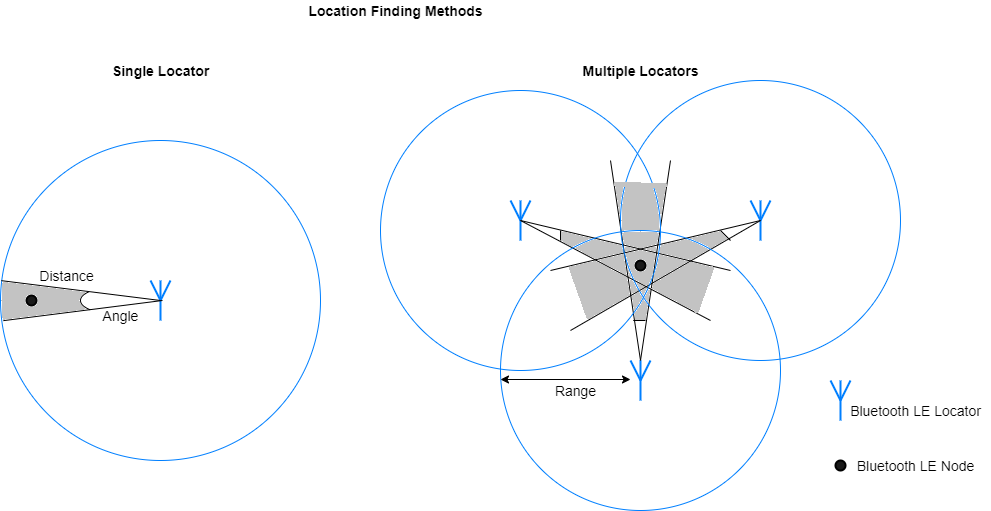• Distance plus angle: If one locator is present in the range of the Bluetooth LE node, use this method to estimate the node position. The location can be estimated by calculating the AoA or AoD of a direction-finding signal and estimating the distance between the locator and node by performing a path loss calculation on the received signal strength indicator (RSSI).

• Angulation: If two or more locators are present in the range of the Bluetooth LE node, use this method to estimate the node position. The location can be estimated by triangulating the AoA or AoD between each locator and node.

### Simulation Parameters

Specify the linear motion model. Generate dynamic node positions by using one of these motion models:

• 2-D constant velocity

• 3-D constant velocity

• 2-D constant acceleration

• 3-D constant acceleration

`motionModel =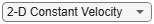"2-D Constant Velocity"; % Linear motion model`

Specify the direction-finding method, the direction-finding packet type, and the physical layer (PHY) transmission mode.

```dfMethod =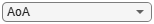"AoA"; % direction-finding method dfPacketType =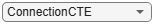"ConnectionCTE"; % direction-finding packet type % PHY transmission mode, must be LE1M or LE2M (for ConnectionCTE) and LE1M % (for ConnectionlessCTE) phyMode =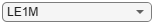"LE1M";```

Specify the antenna array parameters.

```% Antenna array size, must be a scalar (represents ULA) or a vector % (represents URA) for 2-D or 3-D positioning, respectively arraySize =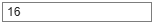16; % Normalized spacing between the antenna elements with respect to % wavelength elementSpacing =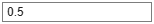0.5; % Antenna switching pattern, must be a 1xM row vector and M must be in the % range [2, 74/slotDuration+1] switchingPattern =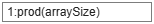1:prod(arraySize);```

Specify the waveform generation and reception parameters.

```slotDuration =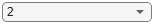2; % Slot duration in microseconds sps =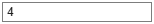4; % Samples per symbol channelIndex =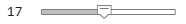17; % Channel index crcInit =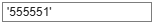'555551'; % Cyclic redundancy check (CRC) initialization accessAddress =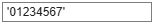'01234567'; % Access address payloadLength =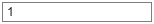1; % Payload length in bytes % Length of constant tone extension (CTE) in microseconds, must be in the % range [16, 160] with 8 microseconds step size cteLength =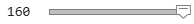160; % Sample offset, must be a positive integer in the range [sps/8,7*sps/8] % for LE1M and [sps/4,7*sps/4] for LE2M sampleOffset =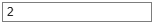2;```

Specify the bit energy to noise density ratio (EbNo), environment and transmitter power.

```EbNo =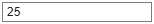25; % Eb/No in dB environment =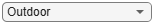"Outdoor"; % Environment txPower =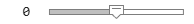0; % Transmit power in dBm```

Validate the simulation parameters.

```% Configure the bluetoothRangeConfig object rangeConfig = bluetoothRangeConfig; rangeConfig.SignalPowerType = "ReceivedSignalPower"; rangeConfig.Environment = environment; rangeConfig.TransmitterPower = txPower; rangeConfig.TransmitterCableLoss = 0; % Transmitter cable loss rangeConfig.ReceiverCableLoss = 0; % Receiver cable loss numDimensions = 2 + (strcmp(motionModel,"3-D Constant Velocity") || strcmp(motionModel,"3-D Constant Acceleration")); if numDimensions == 2 && size(arraySize,2) ~= 1 error("The arraySize must be a scalar for 2-D position estimation"); elseif numDimensions == 3 && size(arraySize,2) ~= 2 error("The arraySize must be a 1-by-2 vector for 3-D position estimation"); end if strcmp(dfPacketType,"ConnectionCTE") && payloadLength ~= 1 error("The payloadLength must be 1 byte for direction-finding packet type of ConnectionCTE"); elseif strcmp(dfPacketType,"ConnectionlessCTE") && payloadLength < 3 error("The payloadLength must be greater than or equal to 3 bytes for direction-finding packet type of ConnectionlessCTE"); end```

Create and configure a Bluetooth LE angle estimation configuration object.

```if numDimensions == 3 && isscalar(elementSpacing) elementSpacing = [elementSpacing elementSpacing]; end cfg = bleAngleEstimateConfig("ArraySize",arraySize, ... "SlotDuration",slotDuration, ... "SwitchingPattern",switchingPattern, ... "ElementSpacing",elementSpacing); validateConfig(cfg); % Validate the configuration object pos = getElementPosition(cfg); % Element positions of an array % Derive type of CTE based on slot duration and direction-finding method if strcmp(dfMethod,"AoA") cteType = [0;0]; else cteType = [0;1]; if slotDuration == 1 cteType = [1;0]; end end```

Create and configure System objects™ for receiver processing.

```% Create and configure preamble detector System object accessAddBitsLen = 32; accessAddBits = int2bit(hex2dec(accessAddress),accessAddBitsLen,false); refSamples = helperBLEReferenceWaveform(phyMode,accessAddBits,sps); prbDet = comm.PreambleDetector(refSamples,"Detections","First"); % Create and configure coarse frequency compensator System object phyFactor = 1+strcmp(phyMode,"LE2M"); sampleRate = 1e6*phyFactor*sps; coarsesync = comm.CoarseFrequencyCompensator("Modulation","OQPSK",... "SampleRate",sampleRate,... "SamplesPerSymbol",2*sps,... "FrequencyResolution",100); % Create and configure CRC detector System object crcLen = 24; % CRC length crcDet = comm.CRCDetector("x^24 + x^10 + x^9 + x^6 + x^4 + x^3 + x + 1","DirectMethod",true,... "InitialConditions",int2bit(hex2dec(crcInit),crcLen).');```

### Packet Transmission and Reception

Perform these steps to track a Bluetooth LE node.

1. Consider 15 locators and a node in a network. Generate 30 node positions based on the motion of the node.

2. At each node position, consider active locators in 80 meters range.

3. Model the direction-finding packet exchange between the node and each active locator. This figure shows the processing chain that the example uses to estimate the angle(s) and distances between the node and active locator.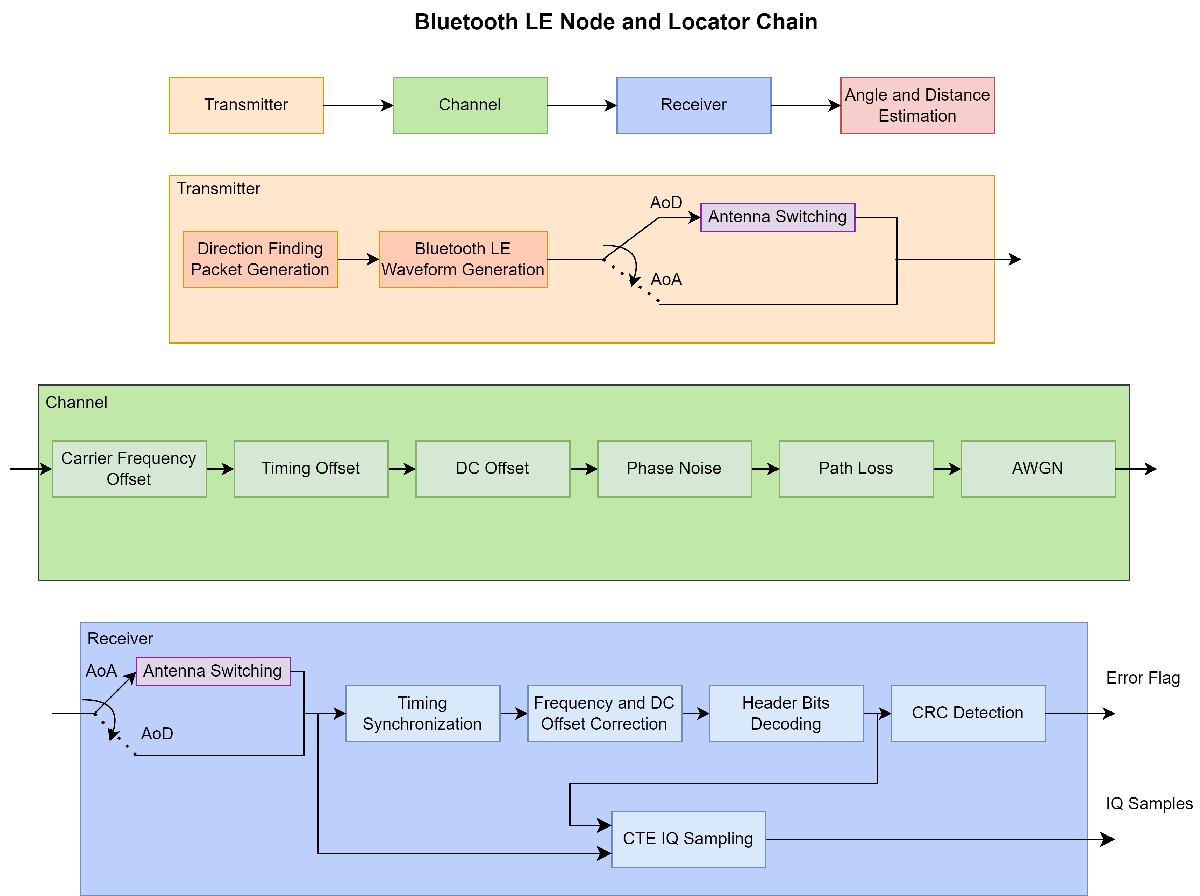4. Repeat steps 2 and 3 for each node position.

5. Estimate the node position by using the known active locator positions, distances, and angles.

```rng('default'); % Initialize the random number generator stream snr = EbNo - 10*log10(sps); % Signal to noise ratio (SNR) in dB headerLen = 16+8*strcmp(dfPacketType,"ConnectionCTE"); % Header length preambleLen = 8*phyFactor; % Preamble length % Derive initial state for whitening based on channel index dewhitenStateLen = 6; chanIdxBin = int2bit(channelIndex,dewhitenStateLen).'; initState = [1 chanIdxBin]; dewhiten = bluetoothWhiten(InitialConditions=initState'); % Consider one node and 15 locators in a network. Generate random locator % positions and generate node positions based on the motion model. numLocators = 15; % Number of locators in a network numNodePositions = 30; % Number of node positions in a network [posNode,posLocators] = helperBLEPositions(motionModel,numNodePositions,numLocators); % Simulate motion of the node by looping over the generated node positions. % For each node position, find active locators. posNodeEst = zeros(numDimensions,numNodePositions); % Initialize the seed S = RandStream('mt19937ar','Seed',5489); % Initialize phase frequency system object pfo = comm.PhaseFrequencyOffset(SampleRate=sampleRate); % Initialize phase noise system object phaseNoise = comm.PhaseNoise(Level=[-130 -136],FrequencyOffset=[1e4 1e5],SampleRate=sampleRate); for inumNode = 1:numNodePositions % Consider active locators that are within 80 meters of range to the % node [posActiveLocators,angleActive,distanceActive] = helperBLEActiveLocators(posNode(:,inumNode),posLocators); posLocatorBuffer{:,inumNode} = posActiveLocators; %#ok<SAGROW> numActiveLocators = size(posActiveLocators,2); % Number of active locators % Estimate the pathloss between the transmitter and the receiver based % on the distance and the environment plLin = helperBluetoothEstimatePathLoss(rangeConfig.Environment,distanceActive); angleEst = zeros(numActiveLocators,numDimensions-1); % Estimated angles [pathLossdB,linkFailFlag] = deal(zeros(numActiveLocators,1)); % Estimated path loss and flag to indicate link fail % Loop over number of active locators for i = 1:numActiveLocators ```

#### Transmitter

``` % Generate direction-finding packet data = helperBLEGenerateDFPDU(dfPacketType,cteLength,cteType,payloadLength,crcInit); % Generate Bluetooth LE waveform bleWaveform = bleWaveformGenerator(data,"Mode",phyMode,"SamplesPerSymbol",sps,... "ChannelIndex",channelIndex,"DFPacketType",dfPacketType,"AccessAddress",accessAddBits); % If the direction-finding method is AoD, perform antenna switching if strcmp(dfMethod,"AoD") bleWaveform = helperBLESteerSwitchAntenna(bleWaveform,angleActive(i,:),... phyMode,sps,dfPacketType,payloadLength,cfg); end```

#### Channel

Add RF impairments to the waveform. Attenuate the impaired waveform according to the transmitter power and pathloss.

``` % Add frequency offset freqOffset = randsrc(1,1,-10e3:100:10e3,S); pfo.FrequencyOffset = freqOffset; freqWaveform = pfo(bleWaveform); % Release the phase frequency object release(pfo) % Add timing offset timingoff = randsrc(1,1,1:0.2:20,S); timingOffWaveform = helperBLEDelaySignal(freqWaveform,timingoff); % Add DC offset dcMaxValue = max(max(real(timingOffWaveform)),max(imag(timingOffWaveform))); dcValue = (5/100)*randsrc(1,1,(-1:0.05:1)*dcMaxValue)*(sqrt(0.5)+sqrt(0.5)*1i); dcWaveform = timingOffWaveform + dcValue; % Add phase noise noisyWaveform = phaseNoise(dcWaveform); % Release the phase noise object release(phaseNoise); % Apply transmitter power and path loss dBmConverter = 30; txImpairedWaveform = 10^((rangeConfig.TransmitterPower-dBmConverter)/20)*noisyWaveform/plLin(i);```

Perform these steps to recover IQ samples for angle estimation.

1. Perform antenna switching (for AoA)

2. Perform packet detection

4. Perform CRC detection

5. Perform CTE IQ sampling

``` % Perform antenna steering if the direction-finding method is AoA if strcmp(dfMethod,"AoA") steerVec = helperBLESteeringVector(angleActive(i,:),pos); steeredWaveform = txImpairedWaveform .* steerVec.'; else steeredWaveform = txImpairedWaveform; end % Initialize the variables used for receiver processing packetDetect = 0; % Packet detect flag headerFlag = 1; % Header flag to perform header decoding pduCRCFlag = 0; % PDU and CRC flag to perform CRC detection samplingFlag = 0; % CTE IQ sampling flag crcError = 1; % Checksum flag samplesPerFrame = 8*sps; % Samples considered for packet detection samplesPerModule = samplesPerFrame+sps; % Samples considered for header, PDU and CRC, CTE IQ sampling numTimes = ceil(length(timingOffWaveform)/samplesPerFrame)+1; % Divide the waveform as per the samples considered countpacketDetect = 0; % Counter for packet detection moduleStartInd = 0; % Start index for each module % Append zeros to the waveform rxWaveformBuffer = [steeredWaveform;zeros(samplesPerFrame*numTimes-length(steeredWaveform)+samplesPerFrame,size(steeredWaveform,2))]; for j = 1:numTimes % Retrieve the waveform corresponding to each module if (j-1)*samplesPerFrame + moduleStartInd + samplesPerModule <= length(rxWaveformBuffer) rxChunkWaveform = rxWaveformBuffer((j-1)*samplesPerFrame+moduleStartInd+(1:samplesPerModule),:); else rxChunkWaveform = rxWaveformBuffer((j-1)*samplesPerFrame+moduleStartInd+1:end,:); end```

A sampling flag is used to handle antenna switching for AoA.

• If the sampling flag is disabled, use the waveform from the first antenna. Add AWGN to the waveform.

• If the sampling flag is enabled, perform antenna switching (for AoA). Add AWGN to the waveform. Perform IQ sampling on the CTE samples.

``` if ~samplingFlag rxNoisyWaveform = awgn(rxChunkWaveform(:,1),snr,"measured"); % Add AWGN else if strcmp(dfMethod,"AoA") % Perform antenna switching rxSwitchWaveform = helperBLESwitchAntenna(rxChunkWaveform,phyMode,sps,slotDuration,switchingPattern); else rxSwitchWaveform = rxChunkWaveform; end rxNoisyWaveform = awgn(rxSwitchWaveform,snr,"measured"); % Add AWGN % Perform IQ sampling on the CTE cteSamples = rxNoisyWaveform(1:cteTime*8*sps*phyFactor); iqSamples = bleCTEIQSample(cteSamples,"Mode",phyMode,... "SamplesPerSymbol",sps,"SlotDuration",slotDuration,"SampleOffset",sampleOffset); samplingFlag = 0; break; end```

Perform these steps for packet detection:

1. Load the waveform into a buffer to perform preamble detection.

2. Estimate and compensate DC and frequency offsets.

3. Perform Gaussian minimum shift keying (GMSK) demodulation.

4. Compare the decoded access address with the known access address. If these addresses match, the packet is detected.

``` if packetDetect == 0 countpacketDetect = countpacketDetect+1; rcvSigBuffer((countpacketDetect-1)*(samplesPerModule-sps)+(1:samplesPerModule)) = rxNoisyWaveform; if countpacketDetect >= (preambleLen+accessAddBitsLen+headerLen)*sps/samplesPerFrame % Perform timing synchronization [~, dtMt] = prbDet(rcvSigBuffer.'); release(prbDet) prbDet.Threshold = max(dtMt); prbIdx = prbDet(rcvSigBuffer.'); release(prbDet) if prbIdx >=length(refSamples) rcvTrim = rcvSigBuffer(1+prbIdx-length(refSamples):end).'; else rcvTrim = rcvSigBuffer.'; end % Estimate and compensate DC offset estimatedDCOffset = mean(rcvTrim(1:length(refSamples)))-mean(refSamples)*sqrt(var(rcvTrim)); rcvDCFree = rcvTrim-estimatedDCOffset; % Estimate and compensate frequency offset [~,freqoff] = coarsesync(rcvDCFree); release(coarsesync) [rcvFreqOffsetFree,iniFreqState] = helperBLEFrequencyOffset(rcvDCFree,sampleRate,-freqoff); % Perform GMSK demodulation x = rem(length(rcvFreqOffsetFree),sps); if x remsad = sps - x; else remsad = x; end remNumSamples = x; remSamples = rcvFreqOffsetFree(end-remNumSamples+1:end); [demodSoftBits,demodInitPhase] = helperBLEGMSKDemod(rcvFreqOffsetFree(1:end-remNumSamples),phyMode,sps,0); demodBits = demodSoftBits>0; % Check for access address match if length(demodBits) >= preambleLen+accessAddBitsLen accessAddress = int8(demodBits(preambleLen+(1:accessAddBitsLen))>0); decodeData = int8(demodBits(accessAddBitsLen+preambleLen+1:end)>0); if isequal(accessAddBits,accessAddress) packetDetect = 1; samplesPerModule = headerLen*sps-length(decodeData)*sps+remsad+sps; rcvSigBuffer = []; end end end end```

Recover the header bits if the packet is detected.

``` if packetDetect if headerFlag && (length(rxNoisyWaveform) ~= samplesPerFrame+sps || samplesPerModule <= sps) % Remove DC offset rxDCFreeWaveform = rxNoisyWaveform-estimatedDCOffset; % Frequency offset correction [rxNoisyWaveform,iniFreqState] = helperBLEFrequencyOffset(rxDCFreeWaveform,sampleRate,-freqoff,iniFreqState); % Perform GMSK demodulation on the header bits if (length(rxNoisyWaveform) ~= samplesPerFrame) rcvSigHeaderBuffer = [remSamples;rxNoisyWaveform]; [headerSoftBits,demodInitPhase] = helperBLEGMSKDemod(rcvSigHeaderBuffer,phyMode,sps,demodInitPhase); decodeDataHeader = [decodeData;headerSoftBits>0]; elseif samplesPerModule <= 0 decodeDataHeader = decodeData; end % Perform data dewhitening dewhitenedBits = dewhiten(decodeDataHeader); % Extract PDU length pduLenField = double(dewhitenedBits(9:16)); % Second byte of PDU header pduLenInBits = bi2de(pduLenField')*8; % Retrieve CTE time and CTE type information from bits if strcmp(dfPacketType,"ConnectionCTE") [cteTime,cteTypeDec] = helperBLECTEInfoExtract(dewhitenedBits,dfPacketType); % Slot duration based on CTE type if cteTypeDec == 1 || cteTypeDec == 2 slotDuration = cteTypeDec; else slotDuration = cfg.SlotDuration; end end % Update the samples per frame, start index to perform % CRC detection if samplesPerModule moduleStartInd = samplesPerModule-samplesPerFrame-sps; else moduleStartInd = -sps; end samplesPerModule = (pduLenInBits+crcLen-length(dewhitenedBits)+headerLen+1)*sps; pduCRCFlag = 1; % Enable the flag for CRC detection headerFlag = 0; % Disable the header recovery flag rcvSigHeaderVar = rxNoisyWaveform; % Load the waveform for pathloss estimation rxNoisyWaveform = []; end```

Perform CRC detection based on the PDU length recovered from header bits.

``` if pduCRCFlag && ~isempty(rxNoisyWaveform) % Remove DC offset rxDCFreeWaveform = rxNoisyWaveform-estimatedDCOffset; % Frequency offset correction [rxNoisyWaveform,iniFreqState] = helperBLEFrequencyOffset(rxDCFreeWaveform,sampleRate,-freqoff,iniFreqState); % Perform GMSK demodulation on the PDU and CRC bits x = rem(length(rxNoisyWaveform),sps); if x remNumSamples = sps - x; else remNumSamples = x; end demodPDUCRC = helperBLEGMSKDemod([rxNoisyWaveform;zeros(remNumSamples,1)],phyMode,sps,demodInitPhase); % Perform data dewhitening dewhitenedPDUCRC = dewhiten(demodPDUCRC); reset(dewhiten) % Retrieve CTE time and CTE type information from bits headerPDUCRC = [double(dewhitenedBits);dewhitenedPDUCRC]; if strcmp(dfPacketType,"ConnectionlessCTE") [cteTime,cteTypeDec] = helperBLECTEInfoExtract(headerPDUCRC,dfPacketType); % Slot duration based on CTE type if cteTypeDec == 1 || cteTypeDec == 2 slotDuration = cteTypeDec; else slotDuration = cfg.SlotDuration; end end % Perform CRC detection [dfPDU,crcError] = crcDet(headerPDUCRC); if crcError break; end % Update the samples per frame, start index to perform % IQ sampling moduleStartInd = samplesPerModule-samplesPerFrame+moduleStartInd-sps; samplesPerModule = cteTime*8*sps*phyFactor; samplingFlag = 1; % Enable the flag to perform IQ sampling pduCRCFlag = 0; % Disable the PDU and CRC flag rcvSigPDUVar = rxNoisyWaveform; % Load the waveform for pathloss estimation end end end```

#### Estimate Angle and Pathloss

Use the IQ samples from the receiver to estimate the angles and path loss.

Perform angle estimation if both of these conditions meet:

• CRC is detected.

• A minimum number of non-zero IQ samples are present in `iqSamples`.

``` % IQ samples must contain at least eight samples from reference % period and one sample from each antenna refSampleLength = 8; % Reference samples length minIQSamples = refSampleLength+getNumElements(cfg)-1; if ~crcError && length(nonzeros(iqSamples)) >= minIQSamples % If packet detection is successful angleEst(i,:) = bleAngleEstimate(iqSamples,cfg); rangeConfig.ReceivedSignalPower = 10*log10(var([rcvFreqOffsetFree;rcvSigHeaderVar;rcvSigPDUVar]))+30; pathLossdB(i) = pathLoss(rangeConfig); else linkFailFlag(i) = 1; % If packet detection fails, enable the link fail flag end end```

#### Estimate Node Position

Estimate the position of the node in the network by using one of these two methods.

• Angulation: If two or more number of active locators are successfully decoded.

• Distance-angle: If one active locator is successfully decoded.

``` if any(linkFailFlag == 1) idx = find(linkFailFlag == 1); posActiveLocators(:,idx) = []; angleEst(idx,:) = []; pathLossdB(idx) = []; end if (numActiveLocators-nnz(linkFailFlag)) >= 2 && ~all(angleEst(:,1) == angleEst(1,1)) % Estimate the node position by using the angulation method posNodeEst(:,inumNode) = blePositionEstimate(posActiveLocators,"angulation",angleEst.'); elseif (numActiveLocators-nnz(linkFailFlag)) == 1 || (~all(linkFailFlag == 1) && all(angleEst(:,1) == angleEst(1,1))) % Estimate the distance between the transmitter and the receiver % based on the environment range = bluetoothRange(rangeConfig); distanceValues = min(range):max(range); idx = randi(length(distanceValues),1); distanceEst = distanceValues(idx) % Estimate the node position by using the distance-angle method posNodeEst(:,inumNode) = blePositionEstimate(posActiveLocators,"distance-angle",distanceEst.',angleEst.'); else posNodeEst(:,inumNode) = NaN(numDimensions,1); end end```

### Simulation Results

Visualize the Bluetooth LE node tracking.

```posErr = sqrt(sum((posNodeEst-posNode).^2)); % Compute the position error disp(["Positioning error in meters = ", num2str(posErr)])```
``` "Positioning error in meters = " "0.065765 0.26985 0.30627 0.14887 0.23468 0.64657 0.33248 0.14222 0.29012 0.14112 0.44808 0.33305 0.18346 0.37073 0.1291 0.5011 0.35704 0.80523 0.44211 0.13569 0.63661 0.55441 0.20427 0.25834 0.42786 0.2838 0.31126 0.20832 0.30865 0.22604" ```
```if ~all(isnan(posNodeEst(1,:))) helperBLEVisualizeNodeTracking(posLocators,posNode,posLocatorBuffer,posNodeEst) end```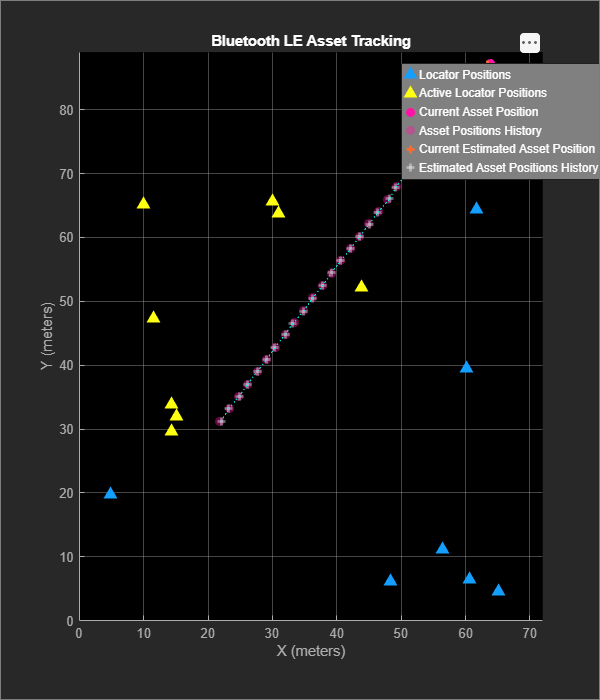This example enables you to estimate the 2-D or 3-D position of a Bluetooth LE node using the direction-finding functionality and location estimation techniques. The example also measures positioning accuracy at each node position.

### Further Exploration

Improve the position accuracy of the Bluetooth LE node by using the Kalman filter from Sensor Fusion and Tracking Toolbox™. The Kalman filter is a recursive algorithm that estimates the track of an object by updating the current state using the previous state. When the evolution of the state follows a linear motion model and the measurements are linear functions of the state, the Kalman filter is linear. For more information about the linear Kalman filter, see Linear Kalman Filters (Sensor Fusion and Tracking Toolbox).

The two important characteristics of Kalman filter are:

• Predicting the node's future location

• Reducing the noise introduced by inaccurate detections

If node estimation measurement is available, the Kalman filter reduces the noise by predicting and correcting together. If node estimation measurement is not available (all the node-locators links fail), the Kalman filter estimates the location based on the previous measurements. As there is no prior knowledge on initial estimates of the velocity or acceleration of node, the position estimation can be transient during the initial phase. Use this sample code snippet after estimating node positions.

```% if all(isnan(posNodeEst(1,:))) % disp("All direction-finding packet transmissions failed") % else % posNodeTrackEst = helperBLEKalmanFilter(motionModel,posNodeEst); % posTrackErr = sqrt(sum((posNodeTrackEst-posNode).^2)); % Compute the position error with Kalman filter % disp(["Positioning error with Kalman filter in meters = ",num2str(posTrackErr)]) % end```

### Appendix

The example uses these helpers:

### Selected Bibliography

1. “Bluetooth® Technology Website – The Official Website for the Bluetooth Wireless Technology. Get up to Date Specifications, News, and Development Info.” Accessed January 4, 2023. https://www.bluetooth.com/

2. “Core Specification – Bluetooth® Technology Website.” Accessed January 4, 2023. https://www.bluetooth.com/specifications/specs/core-specification-5-1/.

3. “Core Specification – Bluetooth® Technology Website.” Accessed January 4, 2023. https://www.bluetooth.com/specifications/specs/core-specification-5-3/.